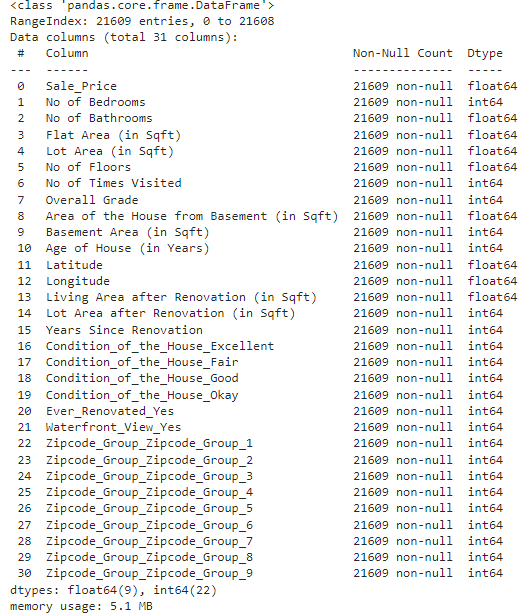top of page
• rishabhdwivedi062

# Data exploration and preprocessing in machine learning - House Price Prediction.

Updated: Oct 6, 2022

Data exploration and preprocessing are the key aspects of machine learning. With proper exploration and preprocessing we can enhance the accuracy of our model to a great extent, which is our ultimate goal.

Let's understand data exploration in parts and solve a real-world problem from scratch.

Problem Statement: House price prediction using a regression model.

You can download the dataset and can open it in google collab to perform the required steps.

Data Exploration and target variable part-1

First, we need to import the necessary python libraries.

```import pandas as pd # Import pandas for playing with the structure.
import numpy as np # Import numpy for numerical analysis

# Read the data set and print first five rows
data.head() # print first 5 rows``````# This will print complete information of the data set, like its type, not # null count, total number of entries etc.

data.info()

``````data['Sale_Price'].head(10) # This will print first 10 rows of sale price
data['Sale_Price'].tail(10) # This will print last 10 rows of sale price``````# describe function will give complete statistical analysis of data set
#which contains information about mean, median, count, min, max etc

data['Sale_Price'].describe()```Hence data exploration part one is completed here, we have seen some basic functions and most importantly the use of describe function.

Data Exploration and target variable part-2

Now we will check the presence of outliers.

Outliers: An outlier is a data point that is distinct from other data points. Its value lies outside the usual range of the rest of the values in the data and hence the term 'outlier'

```# Importing matplotlib and seaborn for visual analysis of dataset.

import matplotlib.pyplot as plt
plt.scatter(x=data['Sale_Price'],y=data['No of Bedrooms'])

# Here we are plotting a scatter plot between sale price column and no of # bedroom ```Here we can see, that there are some points that are not in the same group, hence they are outliers. Let's create a box plot as well.

```import seaborn as sns
# can ignore missing values
sns.boxplot(x=data['Sale_Price'])```Data Exploration and target variable part-3

Different ways of treating outliers:

1) Deletion

2) Capping or Imputation

3) Data Transformation

4) Binning

1--> Deletion: The entire row containing outliers is removed.

Data size is reduced. If dataset is small then valuable information in the process is lost, hence not advisiable to do it.

2-->Impuation: Outlier are not removed but replaced with average/mean/median/mode value

3--> Data Transformation: The variable is transformed to its log value or the square or cube root.

4-->Binning: Different bins are formed based on the values of the variable to treat the outliers.

```q1 = data["Sale_Price"].quantile(0.25) # value at 25%
q3 = data["Sale_Price"].quantile(0.75) # value at 75%

iqr = q3-q1 # difference between them is iqr value
print (iqr)
# Output: 323050.0

upper_limit = q3+1.5*iqr # 1.5 times higher the iqr value
lower_limit = q1-1.5*iqr # 1.5 times lower the iqr value
print(upper_limit,lower_limit)

# Output: (1129575.0, -162625.0)```

Let's make a function and do an imputation, we will use the upper limit and lower limit value. Hence we will replace outliers with those value

```def limit_imputer(value):
if value<lower_limit:
return lower_limit
if value>upper_limit:
return upper_limit
else:
return value```

Let's apply the function on our data set

```data['Sale_Price'] = data['Sale_Price'].apply(limit_imputer)

# describe the function
data['Sale_Price'].describe()```Observation: Mean value is still greater than 50% (Mode) hence anomalies still exist. Data is not normally distributed but a bit skewed towards lower value.

Data Exploration and target variable part-4

Treating missing values.

• Deletion

• Imputation

Entire row containing missing value is reduced.We can loose some important informaion , hence not advisable. A missing value row is not removed but the missing value in it replaced with the mean/average/mode/median value. But it is not advisable to do imputation for target value.(Deletion is prefered)

```data.dropna(inplace=True,axis=0,subset=['Sale_Price'])
# we can perform deletion of missing value using the above code, but since #our dataset does not contains any missing value of dataset will remain # same.```

```# Plot a histogram
plt.hist(data["Sale_Price"],bins=10,color="blue")
plt.xlabel("Interval")
plt.ylabel("Selling Price")
plt.title("Histogram of a selling price")
plt.show()```Data is skewed towards lower value.

Data Exploration: Independent values, part-1

```numerical_columns = ['No of Bedrooms','No of Bathrooms','Flat Area (in Sqft)']

from sklearn.impute import SimpleImputer
imputer = SimpleImputer(missing_values=np.nan,strategy='median')
data[numerical_columns] = imputer.fit_transform(data[numerical_columns])```

Fit: The function calculates the median value with respect to every column that we have passed in as a parameter and stores it

Transform: The function does the actual action of locating the missing values and imputing them using the median strategy.

Sklearn library contains an imputer function.

That's all for this post, in the following post we will do data exploration containing missing values and outliers.